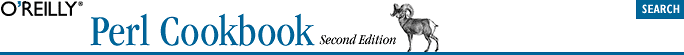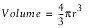home | O'Reilly's CD bookshelfs | FreeBSD | Linux | Cisco | Cisco Exam## 11.6. Creating Arrays of Scalar References

### 11.6.3. Discussion

In the following examples, @array is a simple array containing references to scalars (an array of references is not a reference to an array). To access the original data indirectly, braces are mandatory.

```(\$a, \$b, \$c, \$d) = (1 .. 4);        # initialize
@array =  (\\$a, \\$b, \\$c, \\$d);     # refs to each scalar
@array = \( \$a,  \$b,  \$c,  \$d);     # same thing!
@array = map { \my \$anon } 0 .. 3;  # allocate 4 anon scalar refs

\${ \$array } += 9;                # \$c now 12

\${ \$array[ \$#array ] } *= 5;        # \$d now 20
\${ \$array[-1] }        *= 5;        # same; \$d now 100

\$tmp   = \$array[-1];                # using temporary
\$\$tmp *= 5;                         # \$d now 500```

The two assignments to @array are equivalent—the backslash operator is distributive across a list. So preceding a list (including a slice or a function's return list, but not an array) with a backslash is the same as applying a backslash to everything in that list. The ensuing code changes the values of the variables whose references were stored in the array.

Here's how to deal with such an array without explicit indexing:

```use Math::Trig qw(pi);              # load the constant pi
foreach \$sref (@array) {            # prepare to change \$a,\$b,\$c,\$d
(\$\$sref **= 3) *= (4/3 * pi);   # replace with spherical volumes
}```

This code uses the formula for deriving the volume of a sphere:The \$sref loop index variable is each reference in @array, and \$\$sref is the number itself, that is, the original variables \$a, \$b, \$c, and \$d. Changing \$\$sref in the loop changes those variables as well. First we replace \$\$sref with its cube, then multiply the resulting value by 4/3π. This takes advantage of the fact that assignment in Perl returns an lvalue, letting you chain assignment operators together as we've done using the **= and *= assignment operators.

Actually, anonymous scalars are pretty useless, given that a scalar value fits in the same space as a scalar reference. That's why there's no explicit composer. Scalar references exist only to allow aliasing—which can be done in other ways.